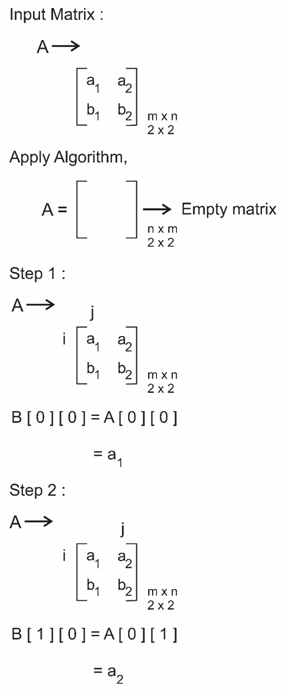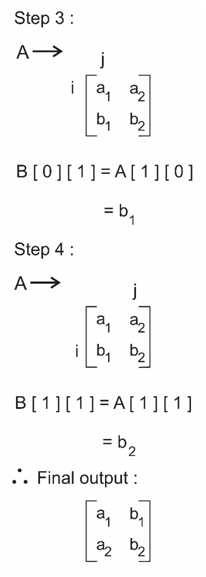# Transpose of a Matrix

## Given two matrices, we need to add the two matrices and give new matrix.

Transpose of a matrix :  The transpose of a matrix is a new matrix whose rows are the columns and columns are the rows of the original.

### Example

Input : A = { {1, 4, 7, 9},
{1, 6, 7, 6},
{6, 3, 7, 2},
{4, 4, 4, 4}}

Output matrix will be :
{ {1, 1, 6, 4},
{4, 6, 3, 4},
{7, 7, 7, 4},
{9, 6, 2, 4}}

## Algorithm

Step 1 : Create an auxiliary dummy matrix which stores the transpose of the matrix.
Step 2 : For a row in the first matrix, make it as first column in new constructed matrix.
Step 3 : move to next row and do it for all the rows.
B[i][j] = A[j][i] , B is Transpose of A
Step 4 : After completing all the rows, print the new matrix, it is Transpose of the given matrix.

### Algorithm working## C++ Program

``````#include <bits/stdc++.h>
using namespace std;
#define N 4
int main()
{
int A[N][N] = { {1, 4, 7, 9},
{1, 6, 7, 6},
{6, 3, 7, 12},
{4, 4, 4, 4}};

int B[N][N] ;

for(int i=0; i <N; i++)
{
for(int j=0; j<N; j++)
{
B[i][j] = A[j][i];
}
}

for(int i=0;i<N;i++)
{
for(int j=0;j<N;j++)
cout<<B[i][j]<<" ";
cout<<endl;
}

return 0;
}``````

Scroll to Top